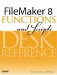# Ln()

Category: Number

 Syntax: Ln ( number )

Parameters:

numberAny positive number or expression that returns a positive number.

Data type returned: Number

Description:

Returns the natural logarithm of the specified number. The natural logarithm uses the transcendental number e as its base. The value of e is approximately 2.71828.

Exp() and Ln() are inverse functions of one another.

The Log() and Lg() functions produce base-10 and base-2 logarithms, respectively. The Ln() function produces base-e logarithms, but it can also be used to solve a logarithm of any base. Log (base-x) of y is equivalent to Ln (y) / Ln (x).

Examples:

Function

Results

Ln (2.7182818)

Returns .9999999895305023.

Ln (Exp(1))

Returns 1.

Ln (100)

Returns 4.6051701859880914.

Ln (Exp(5))

Returns 5.FileMaker 8 Functions and Scripts Desk Reference
ISBN: 0789735113
EAN: 2147483647
Year: 2004
Pages: 352